cancel
Showing results for
Did you mean:Frequent Visitor

## Counting Text Values Across Multiple Dynamic Columns by User

Hi

I have a Matrix Table as below, I want to count the total number of learning activitys then work out the completion percentage, for example here there are 7 learning outcomes in total and line 1 (User dp0m6gp) has completed 4 of these so I want to show this as a percentage and so on for each user ?

I also have a slicer that shows different training programmes that have more or less training outcomes therfore the number of learning outcomes increases or decreases for each programme.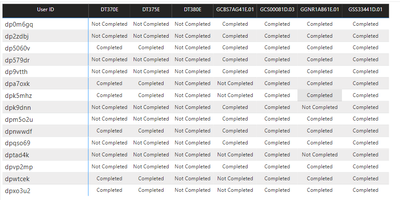2 ACCEPTED SOLUTIONSSuper User

Hi @CalexUK
Should it be something like this?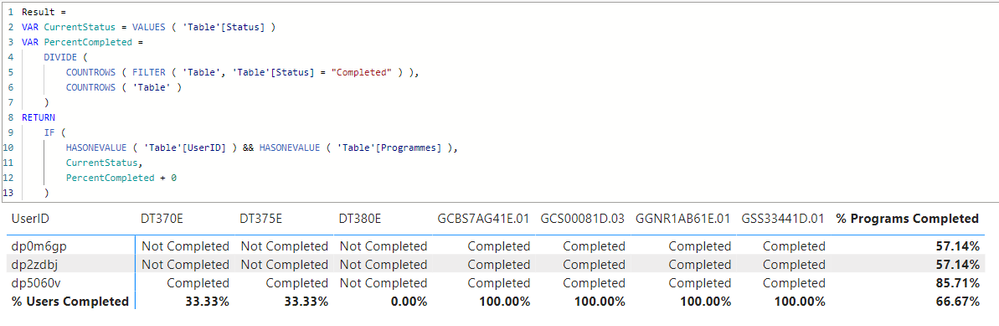``````Result =
VAR CurrentStatus = VALUES ( 'Table'[Status] )
VAR PercentCompleted =
DIVIDE (
COUNTROWS ( FILTER ( 'Table', 'Table'[Status] = "Completed" ) ),
COUNTROWS ( 'Table' )
)
RETURN
IF (
HASONEVALUE ( 'Table'[UserID] ) && HASONEVALUE ( 'Table'[Programmes] ),
CurrentStatus,
PercentCompleted + 0
)``````Super User

@CalexUK
Perhaps somthing like "< Parameter" makes sense?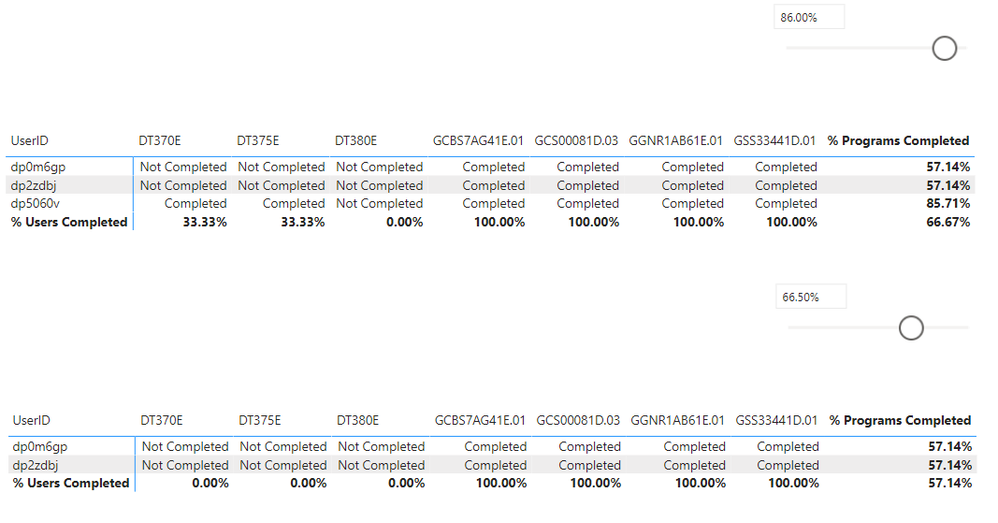7 REPLIES 7Community Support

Hi  @CalexUK ,

Here are the steps you can follow：

1. Create calculated table.

``````Table 2 =
var _summarize =
SUMMARIZE(
'Table',
[User ID],
"training programmes","completed %")
var _table=
SUMMARIZE(
'Table','Table'[User ID],'Table'[training programmes])
return
UNION(
_table,_summarize)``````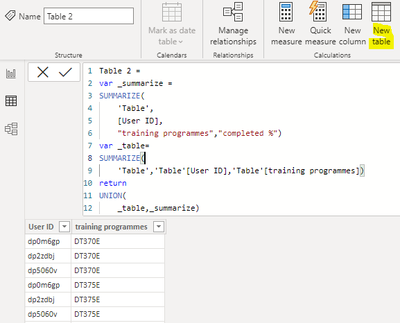2. Create measure.

``````Flag_1 =
MAXX(
FILTER(ALL('Table'),
'Table'[User ID]=MAX('Table 2'[User ID])&&'Table'[training programmes]=MAX('Table 2'[training programmes])),[Measure])``````
``````Flag_2 =
IF(
MAX('Table 2'[training programmes]) ="completed %",
DIVIDE(
COUNTX(
FILTER(ALLSELECTED('Table 2'),'Table 2'[User ID]=MAX('Table 2'[User ID])&&
[Flag_1]="Completed"),[User ID]),
COUNTX(
FILTER(ALLSELECTED('Table 2'),'Table 2'[User ID]=MAX('Table 2'[User ID])),[User ID])-1),
[Flag_1])``````

3. Result: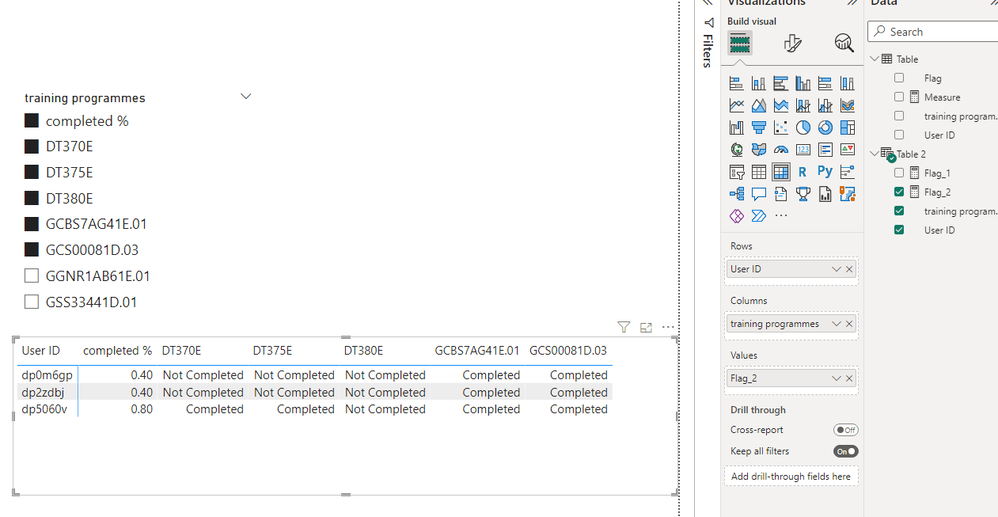Best Regards,

Liu Yang

If this post helps, then please consider Accept it as the solution to help the other members find it more quicklySuper User

Hi @CalexUK

is everything is one source table? What is the dax measure code that is placed in the values of the matrix?Frequent Visitor

Hi Tamerj1

The Data is in a single table, there is no DAX measure, we are only using the Matris Visual to display the training course values (Completed, Not Completed)

Kindest Regards

PaulSuper User

Hi @CalexUK
Should it be something like this?``````Result =
VAR CurrentStatus = VALUES ( 'Table'[Status] )
VAR PercentCompleted =
DIVIDE (
COUNTROWS ( FILTER ( 'Table', 'Table'[Status] = "Completed" ) ),
COUNTROWS ( 'Table' )
)
RETURN
IF (
HASONEVALUE ( 'Table'[UserID] ) && HASONEVALUE ( 'Table'[Programmes] ),
CurrentStatus,
PercentCompleted + 0
)``````Frequent Visitor

Thank you this worked perfect...Frequent Visitor

Hi Tamerj!

This solution worked great, is there a way to add a filter to this measure so that I can filter out whos is at say 100% for all items ? or 50% etc ?

PaulSuper User

@CalexUK
Perhaps somthing like "< Parameter" makes sense?